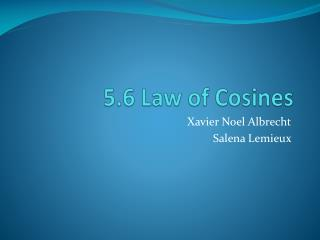DownloadDownload Presentation5.6 Law of Cosines

# 5.6 Law of Cosines

Download Presentation## 5.6 Law of Cosines

- - - - - - - - - - - - - - - - - - - - - - - - - - - E N D - - - - - - - - - - - - - - - - - - - - - - - - - - -

1. ### 5.6 Law of Cosines

Xavier Noel Albrecht Salena Lemieux
2. Introduction
3. Explanation Law of Cosine— a2=b2+c2-2bcCosA b2=a2+c2-2acCosB c2=a2+b2-2abCosC
4. Explanation First you need to identify what you have You need to have three sides (SSS) OR two sides and an angle in between them (SAS) Put the information that you have and put it into the correct formula Solve for the missing side or angle
5. Example #1- Finding a side a² = b² + c² −2(a)(b)cos(A°) a² = 20² + 13² −2(20)(13)cos(66°) a² = 400 + 169 −520cos(66°) a² = 569 −211 a² = 358 a = 3581/2 a = 18.9
6. Example #2-Finding a side a² = b² + c² −2(b)(c)cos(A°) a² = 52² + 16² −2(52)(16)cos(115°) a² = 2,704 + 256 − 1,664cos(115°) a² = 2,960− 1,664(-.423) a² = 2,960 +703 a² = 3663 a=36631/2 a=60.5
7. Example #3 Finding an angle a² = b² + c² −2(a)(b)cos(A°) 14² = 20 ² + 12² −2(12)(20)cos(A°) 14²- 20 ² - 12² = (12)(20)cos(A°) -348=-480cos(A°) 0.675=cos(A°) cos-1(0.25) Cos(A°)=47.5°
8. Example #4- Finding an angle a² = b² + c² −2(a)(b)cos(A°) 25² = 32² + 37² −2(32)(37)cos(A°) 625 = 2393 − 2,368 cos(A°) -1760=-2,368cos(A°) 0.7432=cos(A°) cos-1(0.7432) Cos(A°)=42°
9. Checking The longest side has the largest angle and the smallest side has the smallest angle This won’t give you the exact answer but it will help see if you have the right idea
10. Activity http://quizlet.com/16377121/law-of-sines-and-law-of-cosines-flash-cards/ Who ever can get the most right wins
11. Assessment a=12 b=21 C=95° Find side c. A)23.261 B)30 C)162.32 a=3 b=7 c=6 Find angle B° A)126.83° B)90° C)83.621° c=8 B=131 a=13 Find side b A)53 B)9.825 C)528.9 a=27 b=19 c=24 Find angle A° A)70° B)76.817° C)71.867° A=55° b=12 c=7 Find side a A)3.83 B)15 C)9.831 A=55° b=14 c=23 Find side a A)18.858 B)20 C)16.252 C=42° b=12 a=14 Find side c A)9.503 B)15 C)30.59 A=82° b=22 c=31 Find side a A)40 B)82.453 C)35.428 C=65° a=19 b=22 Find side c A)50 B)22.174 C)47.122 B=31° a=8 c=11 Find side b A)5.843 B)3.485 C)12
12. Work Cited http://www.mathwarehouse.com/trigonometry/law-of-cosines-formula-examples.php http://quizlet.com/16377121/law-of-sines-and-law-of-cosines-flash-cards/ Notes from class Problems from book